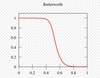《无线电工程》

Butterworth filter

• 所需频率为 1kHz 和 1.7kHz。 它添加了高斯白噪声。 嘈杂的信号被带通过滤，然后是带阻。 滤波器巴特沃斯二阶设计，通过增加阶数会更好。 随意尝试，您也可以插入自己的信号。matlab
• labview设计的巴特沃斯滤波器，参数可自由选择。读取TXT文件数据，显示滤波先后的波形及频谱。labview代码中结合了matlab代码
• 该文件包括使用双线性变换设计巴特沃斯滤波器在哪里Ap = 通带衰减As =阻带衰减PCF = 通带角频率SCF = 阻带角频率是通过双线性变换设计巴特沃斯滤波器的给定参数。 文件还包括1) 得到的传递函数的频率图2) 零极点图matlab
• 基于LabVIEW的巴特沃斯滤波器设计............................................................................................................................................................................滤波器
• 巴特沃斯滤波器（完成） ＃＃要求 Python 2.7 或更高版本（使用 Python 2.7.8 测试） python-opencv（使用 opencv 2.4.4 测试） matplotlib（用 matplolib 1.4.3 测试） ＃＃谢谢 是一个很好的 Gabor 过滤工具...Python
• 该文档包含了巴特沃斯滤波器、切比雪夫1、2滤波器以及椭圆函数形滤波器分别在低通、高通、帯通、帯阻的matlab代码实现。
• 用matlab m文件实现巴特沃斯滤波器，内附有详细的代码说明，和参数修改位置，根据个人需要可以随意设置参数
• labview设计的巴特沃斯滤波器，参数可自由选择。读取TXT文件数据，显示滤波先后的波形及频谱。labview代码中结合了matlab代码
• 一个基于MATLAB的巴特沃斯滤波器设计，有需自取巴特沃斯滤波器
• 在这个程序中，我为低通配置的巴特沃斯滤波器进行编程，这里我们需要通带边缘频率、阻带边缘频率、通带边缘和阻带衰减。 我们得到它的频率响应和零极点图。matlab
• 数字信号处理课程设计 日25 年 6 月2015 1 目 录 一设计目的 .3 二设计要求 .3 三设计内容 .4 3.1选择巴特涡斯低通数据滤波器及双线性变换法的原因 .4 3.2巴特沃思低通滤波器的基本原理 .4 3.3双线性变换法原理 .
•滤波器
• 该文档较为详细地总结了巴特沃斯滤波器的设计，其中包括二阶、三阶巴特沃斯滤波器的设计。
• 数字图像处理，评语滤波，理想低通滤波器，高斯滤波器，巴特沃斯滤波器数字图像处理
• IIR巴特沃斯滤波器实现（内含完整的MATLAB代码、CCS3.3软件仿真代码以及DSP实习报告）
• 本文件为基于LabVIEW的巴特沃斯滤波器设计，无错误，可直接运行。
•matlab
• 如果您不想在每次过滤信号时都经历设计和实现具有归一化频率的滤波器的繁琐程序，那么这个包可能适合您。 如果您是 Matlab 专家和数字信号处理专家，您可能不会对此印象深刻。 每个函数都采用 [filtered_signal,...matlab
• 巴特沃斯滤波器详解

千次阅读 2021-11-25 09:50:44
巴特沃斯滤波器 这里不再赘述低通、高通、带通、带阻滤波器等 巴特沃斯（Butterworth）滤波器在现代设计方法设计的滤波器中，是最为有名的滤波器，由于它设计简单，性能方面又没有明显的缺点，又因它对构成滤波器...

巴特沃斯滤波器

这里不再赘述低通、高通、带通、带阻滤波器等

巴特沃斯（Butterworth）滤波器在现代设计方法设计的滤波器中，是最为有名的滤波器，由于它设计简单，性能方面又没有明显的缺点，又因它对构成滤波器的元件Q值较低，因而易于制作且达到设计性能，因而得到了广泛应用。其中，巴特沃斯滤波器的特点是通频带的频率响应曲线最平滑。

巴特沃斯滤波器传递函数

巴特沃斯低通滤波器可用如下振幅的平方对频率的公式表示：
∣ H ( ω ) ∣ 2 = 1 1 + ( ω ω c ) 2 n = 1 1 + ϵ 2 ( ω ω p ) 2 n |H(\omega)|^2 = \frac{1}{1+(\frac{\omega}{\omega_c})^{2n}} = \frac{1}{1+\epsilon^2(\frac{\omega}{\omega_p})^{2n}}
其中， n n 为滤波器的阶数， ω c \omega_c 为截至频率，即振幅下降为 − 3 d B -3dB 时的频率， ω p \omega_p 为通频带边缘频率。

下图为巴特沃斯滤波器的频率响应图n − > ∞ n->\infty 时，得到一个理想的低通滤波反馈: ω ω c < 1 \frac{\omega}{\omega_c} < 1 时，增益为1； ω ω c > 1 \frac{\omega}{\omega_c} > 1 时，增益为1； ω ω c = 1 \frac{\omega}{\omega_c} = 1 时，增益为0.707

巴特沃斯滤波器的特点是通频带内的频率响应曲线最大限度平坦，没有起伏，而在阻频带则逐渐下降为零。 在振幅的对数对角频率的波特图上，从某一边界角频率开始，振幅随着角频率的增加而逐步减少，趋向负无穷大。巴特沃斯滤波器的频率特性曲线，无论在通带内还是阻带内都是频率的单调函数。因此，当通带的边界处满足指标要求时，通带内肯定会有裕量。所以，更有效的设计方法应该是将精确度均匀的分布在整个通带或阻带内，或者同时分布在两者之内。这样就可用较低阶数的系统满足要求。这可通过选择具有等波纹特性的逼近函数来达到。

python代码

from scipy import signal

1、低通滤波

这里假设采样频率为1000hz,信号本身最大的频率为500hz，要滤除400hz以上频率成分，即截至频率为400hz,则wn=2*400/1000=0.8。Wn=0.8

b, a = signal.butter(8, 0.8, 'lowpass')   #配置滤波器 8 表示滤波器的阶数
filtedData = signal.filtfilt(b, a, data)  #data为要过滤的信号

2、高通滤波

这里假设采样频率为1000hz,信号本身最大的频率为500hz，要滤除100hz以下频率成分，即截至频率为100hz,则wn=2*100/1000=0.2。Wn=0.2

b, a = signal.butter(8, 0.2, 'highpass')   #配置滤波器 8 表示滤波器的阶数
filtedData = signal.filtfilt(b, a, data)  #data为要过滤的信号

3、带通滤波

这里假设采样频率为1000hz,信号本身最大的频率为500hz，要滤除100hz以下，400hz以上频率成分，即截至频率为100，400hz,则wn1=2100/1000=0.2，Wn1=0.2； wn2=2400/1000=0.8，Wn2=0.8。Wn=[0.02,0.8]

b, a = signal.butter(8, [0.2,0.8], 'bandpass')   #配置滤波器 8 表示滤波器的阶数
filtedData = signal.filtfilt(b, a, data)  #data为要过滤的信号

4、带阻滤波

这里假设采样频率为1000hz,信号本身最大的频率为500hz，要滤除100hz以上，400hz以下频率成分，即截至频率为100，400hz,则wn1=2100/1000=0.2，Wn1=0.2； wn2=2400/1000=0.8，Wn2=0.8。Wn=[0.02,0.8]，和带通相似，但是带通是保留中间，而带阻是去除。

b, a = signal.butter(8, [0.2,0.8], 'bandstop')   #配置滤波器 8 表示滤波器的阶数
filtedData = signal.filtfilt(b, a, data)  #data为要过滤的信号

函数介绍

输入参数：
b: 滤波器的分子系数向量
a: 滤波器的分母系数向量
x: 要过滤的数据数组。（array型）
axis: 指定要过滤的数据数组x的轴
irlen：当method为“gust”时，irlen指定滤波器的脉冲响应的长度。如果irlen是None，则脉冲响应的任何部分都被忽略。对于长信号，指定irlen可以显著改善滤波器的性能。（int型或None）

输出参数：
y:滤波后的数据数组

scipy.signal.butter(N, Wn, btype='low', analog=False, output='ba')

输入参数：
N:滤波器的阶数
Wn：归一化截止频率。计算公式Wn=2*截止频率/采样频率。（注意：根据采样定理，采样频率要大于两倍的信号本身最大的频率，才能还原信号。截止频率一定小于信号本身最大的频率，所以Wn一定在0和1之间）。当构造带通滤波器或者带阻滤波器时，Wn为长度为2的列表。
btype : 滤波器类型{‘lowpass’, ‘highpass’, ‘bandpass’, ‘bandstop’},
output : 输出类型{‘ba’, ‘zpk’, ‘sos’}

输出参数：
b，a: IIR滤波器的分子（b）和分母（a）多项式系数向量。output='ba'
z,p,k: IIR滤波器传递函数的零点、极点和系统增益. output= 'zpk'
sos: IIR滤波器的二阶截面表示。output= 'sos'

最后附上matlab实现代码

fs = 1000 ; %信号的采样频率
Rp=1;           %通带最大波纹度 ，单位dB (不要太小)
Rs=30;          %表示阻带最小衰减，单位dB
[N,Wn]=buttord(wp,ws,Rp,Rs);%巴特沃斯数字滤波器的阶数n和-3dB归一化截止频率Wn

[B,A]=butter(N,Wn);%得到n阶巴特沃斯滤波的分子分母
dataOut = filter(B,A,dataIn);

展开全文信号处理 算法 嵌入式
• 巴特沃斯滤波器原理及其仿真设计

千次阅读 热门讨论 2021-09-16 20:03:44
为了进一步探究滤波这条知识线路，今天对巴特沃斯滤波器滤波器进行了研究。 1、什么是巴特沃斯滤波器？ 巴特沃斯（Butterworth）滤波器是一种具有最大平坦幅度响应的低通滤波器，它在通信领域里已有广泛应用，在电...

前面的几篇文章对一阶低通滤波器的原理及其数字化进行了探究。为了进一步探究滤波这条知识线路，今天对巴特沃斯滤波器滤波器进行了研究。

1、什么是巴特沃斯滤波器？

巴特沃斯（Butterworth）滤波器是一种具有最大平坦幅度响应的低通滤波器，它在通信领域里已有广泛应用，在电测中也具有广泛的用途，可以作检测信号的滤波器。文献［张殿龙 王福文．巴特沃斯低通滤波器在电动机测试中的应用［J］成功地将巴特沃斯低通滤波器应用于电动机测试中。 补充一下通带频率和阻带频率的概念，能够通过滤波器的频率信号范围构成通带（pass-bands）,而被衰减的频率信号则不能在输出端输出，这些被衰减的频率范围构成了滤波器的阻带（stop-bands）。通带与阻带交界点的频率称为截止频率。理想的滤波器其频率响应于通带区没有插入损耗(insertion-loss)，而于阻带则有无限大衰减以阻绝讯号通过。然而实际电路无法同时满足上述要求，故在设计电路时应选择适当滤波器种类以符合所需的规格。

2、巴特沃斯滤波器的特点

（1）最大平坦性。幅频特性的前２ｎ－１阶导数均为零，因此在截止频率前较为平坦，这个平坦也保证了信号的原始值，不会因为滤波被衰减。巴特沃斯低通滤波器的通频带最大扁平效应使通频带的增益得到扁平优化.

（2）幅频特性是单调下降，相频特性也是单调下降。巴特沃斯低通滤波器是全极点滤波器，所有零点都在无穷远处；它的幅度随频率的增加而单调下降，且频率无穷大时它的幅度才衰减完

（3）无论阶数ｎ是什么数，都会通过［ω＝ωｂ，｜Ｇ（ｊω）｜＝１／槡２］点。而且ｎ越大，其幅频响应就越逼近理想情况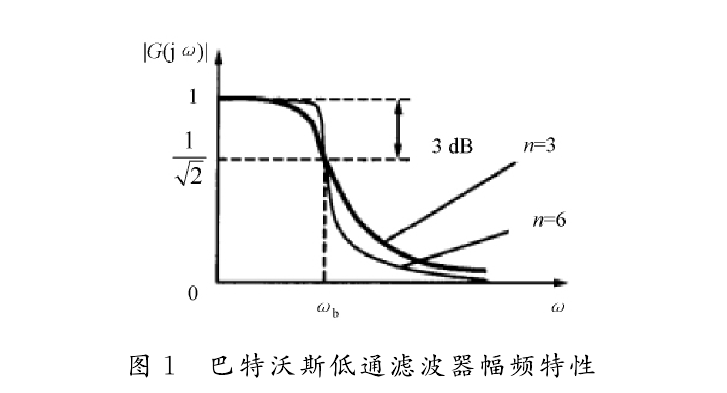3、巴特沃斯滤波器的设计

在了解基本概念后，如何设计巴特沃斯滤波器滤波器是最关键的。对于巴特沃斯滤波器而言，n 阶巴特沃斯低通滤波器的振幅和频率关系可用如下的公式表示: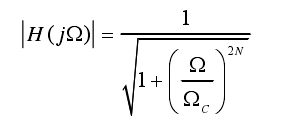而巴特沃斯低通滤波器将其写为传递函数的形式，可以写为：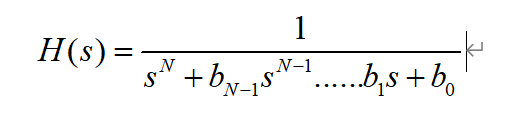如果根据需要的截止频率、滤波器的阶数计算出上式中的所有系数b，即可完成对巴特沃斯滤波器的设计。以前其实是需要很完整的推导和计算的，现在matlab的实时更新对于巴特沃斯滤波器的工具箱进行充盈，在此记录一些工具函数的用法。

1、[N,wn]=buttord(wp,ws,rp,rs,'s')  buttord函数

此函数的功能是根据数字滤波器的通带、阻带截止频率、通带衰减倍数、阻带衰减倍数来计算巴特沃斯数字滤波器的阶数N和3dB截止频率wn。

其中，调用参数wp，ws分别为数字滤波器的通带、阻带截止频率，当ws≤wp时，为高通滤波器；当wp和ws为二元矢量时，为带通或带阻滤波器，这时w n 也是二元向量。rp，rs分别为通带最大衰减和组带最小衰减（dB）。

2 [B，A]=butter（N，wn‘，ftype’butter 函数

此函数的功能是根据滤波器阶数N和截止频率 wn计算N阶巴特沃斯数字滤波器系统函数分子、分母多项式的系数向量B、A。其中，调用参数N和wn分别为巴特沃斯数字滤波器的阶数和3 d B 截止频率，一般是可与buttord格式计算N和wn配合使用。系数B、A是按照z-1的升幂排列，ftype为滤波器的类型。N，wn为butter函数的调用参数。

3 buttap 函数 [Z，P，K] = buttap(N)

函数可设计出N阶巴特沃斯低通滤波器的零、极点。

N = 2;
wc = 100;
[B,A]= butter(N,wc,'s');  %代入N和wn设计低通巴特沃斯模拟滤波器
x = B
y = A
grid;

计算得出：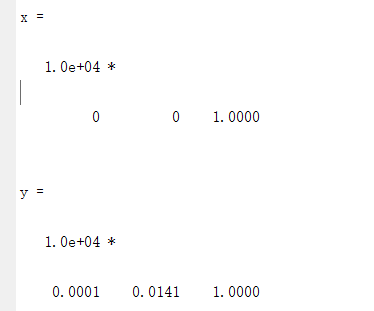因此，此时可以得到传递函数的表达式如下所示：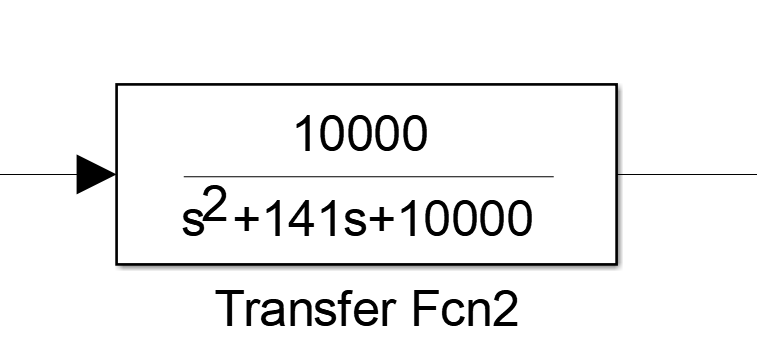4、巴特沃斯滤波器的性能测试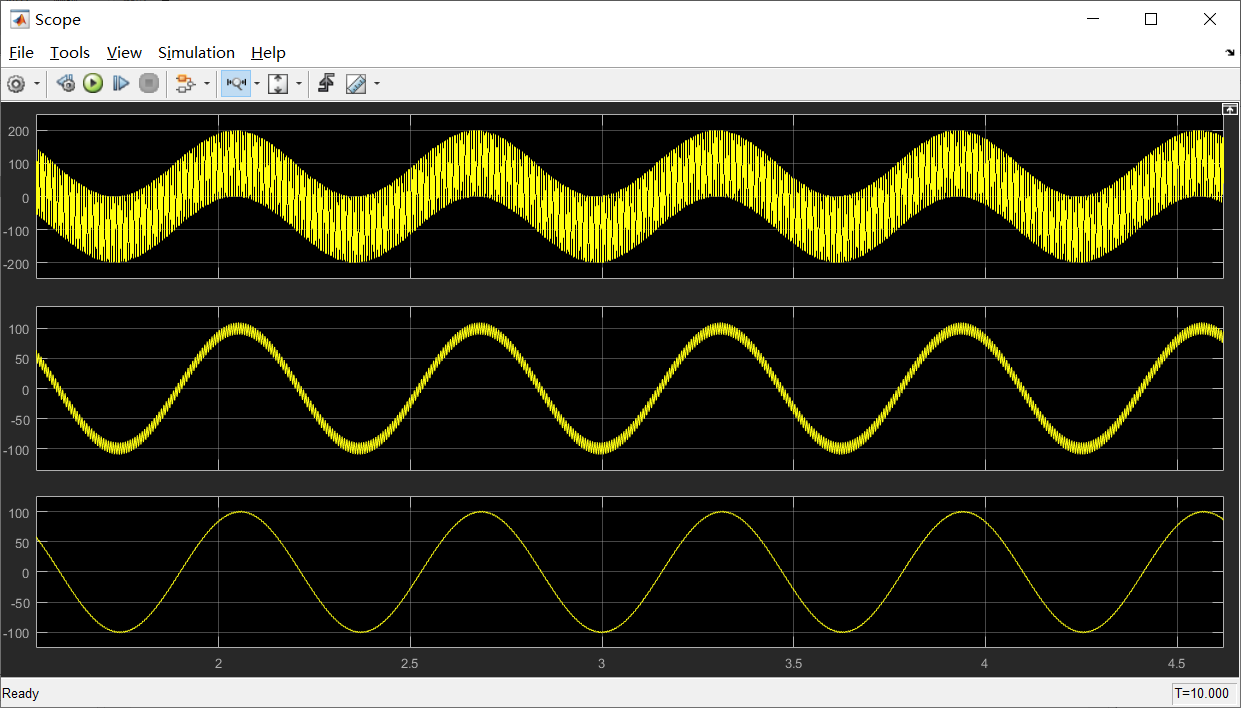接下来对两种低通滤波器的bode图进行分析，如下图所示，可以从图中看出两者的截止频率完全一致，但是在截止频率后的衰减巴特沃斯滤波器明显要比一阶低通滤波器更大，所以在稳态裕度允许的条件下，巴特沃斯滤波器性能更优。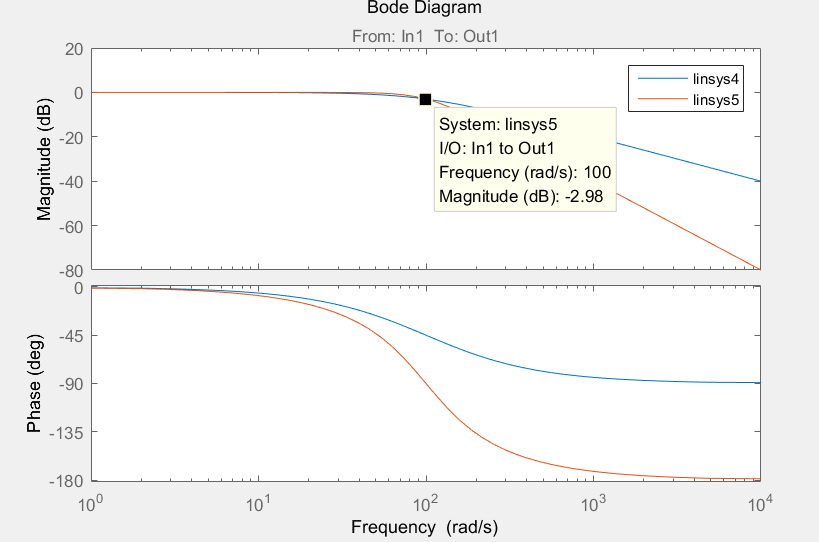小结：

1、巴特沃斯（Butterworth）滤波器是一种具有最大平坦幅度响应的低通滤波器，保证了信号的原始值，不会因为滤波被衰减。

2、巴特沃斯滤波器的性能明显优于一阶低通滤波器。

3、ｎ越大，其幅频响应就越逼近理想情况。（这个还未做，有兴趣的同学可以在我的基础上往下深入探究一下）

4、如果需要巴特沃斯滤波器模型的同学同志，可以在评论区留下你们的邮箱，我看到就会发过去。

展开全文巴特沃斯滤波器 低通滤波器 电机控制 pmsm
• 什么是巴特沃斯滤波器巴特沃斯滤波器是电子滤波器的一种。巴特沃斯滤波器的特点是通频带的频率响应曲线最平滑。这种滤波器最先由英国工程师斯替芬·巴特沃斯(StephenButterworth)在1930年发表在英国《无线电工程》...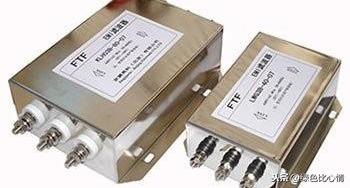什么是巴特沃斯滤波器

巴特沃斯滤波器是电子滤波器的一种。巴特沃斯滤波器的特点是通频带的频率响应曲线最平滑。这种滤波器最先由英国工程师斯替芬·巴特沃斯(StephenButterworth)在1930年发表在英国《无线电工程》期刊的一篇论文中提出的。巴特沃斯滤波器的振幅对角频率单调下降，并且也是唯一的无论阶数，振幅对角频率曲线都保持同样的形状的滤波器。巴特沃斯滤波器的特点是通频带内的频率响应曲线最大限度平坦，没有起伏，而在阻频带则逐渐下降为零。二阶巴特沃斯滤波器的衰减率为每倍频12分贝、三阶巴特沃斯滤波器的衰减率为每倍频18分贝、如此类推。

巴特沃斯滤波器特性

巴特沃斯滤波器的特点是通频带内的频率响应曲线最大限度平坦，没有起伏，而在阻频带则逐渐下降为零。在振幅的对数对角频率的波特图上，从某一边界角频率开始，振幅随着角频率的增加而逐步减少，趋向负无穷大。

一阶巴特沃斯滤波器的衰减率为每倍频6分贝，每十倍频20分贝。二阶巴特沃斯滤波器的衰减率为每倍频12分贝、三阶巴特沃斯滤波器的衰减率为每倍频18分贝、如此类推。巴特沃斯滤波器的振幅对角频。单调下降，并且也是唯一的无论阶数，振幅对角频率曲线都保持同样的形状的滤波器。只不过滤波器阶数越高，在阻频带振幅衰减速度越快。其他滤波器高阶的振幅对角频率图和低级数的振幅对角频率有不同的形状。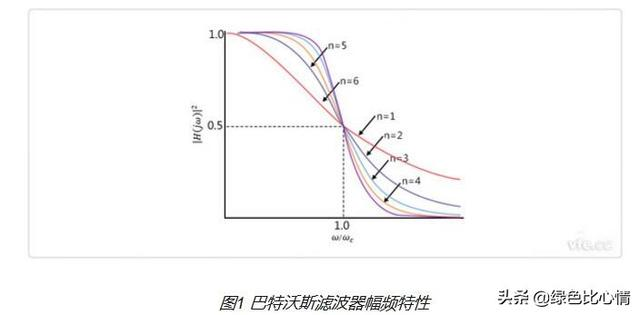巴特沃斯滤波器原理

巴特沃斯型滤波器在现代设计方法设计的滤波器中，是最为有名的滤波器，由于它设计简单，性能方面又没有明显的缺点，又因它对构成滤波器的元件Q值较低，因而易于制作且达到设计性能，因而得到了广泛应用。其中，巴特沃斯滤波器的特点是通频带的频率响应曲线最平滑。

滤波器的截止频率的变换是通过先求出待设计滤波器的截止频率与基准滤波器的截止频率的比值M，再用这个M去除滤波器中的所有元件值来实现的，其计算公式如下：M=待设计滤波器的截止频率/基准滤波器的截止频率。

滤波器的特征阻抗的变换是通过先求出待设计滤波器的特征阻抗与基准滤波器的特征阻抗的比值K，再用这个K去乘基准滤波器中的所有电感元件值和用这个K去除基准滤波器中的所有电容元件值来实现的。

巴特沃斯滤波器与其它滤波器比较

下图是巴特沃斯滤波器(左上)和同阶第一类切比雪夫滤波器(右上)、第二类切比雪夫滤波器(左下)、椭圆函数滤波器(右下)的频率响应图。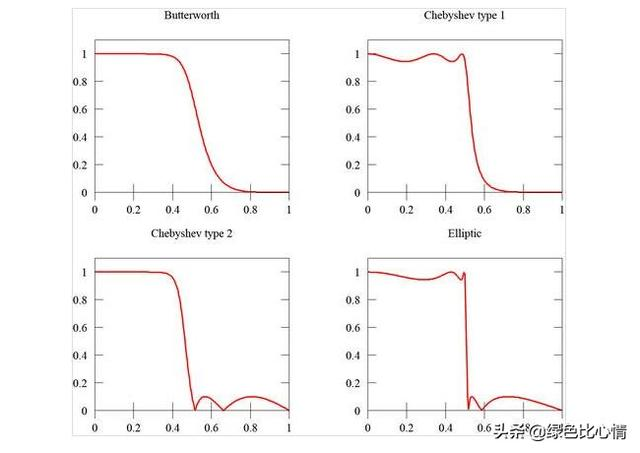特沃斯滤波器优点

巴特沃斯滤波器的特点是通频带内的频率响应曲线最大限度平坦，没有起伏，而在阻频带则逐渐下降为零。在振幅的对数对角频率的波得图上，从某一边界角频率开始，振幅随着角频率的增加而逐渐减少，趋向负无穷大。

一阶巴特沃斯滤波器的衰减率为每倍频6分贝，每十倍频20分贝。二阶巴特沃斯滤波器的衰减率为每倍频12分贝，三阶巴特沃斯滤波器的衰减率为每倍频18分贝，如此类推。巴特沃斯滤波器的振幅对角频率单调下降，并且也是唯一的无论阶数、振幅对角频率曲线都保持同样的形状的滤波器。只不过滤波器阶数越高，在阻频带振幅衰减速度越快。其他滤波器高阶的振幅对角频率图和低级数的振幅对角频率有不同的形状。

巴特沃斯滤波器是滤波器的一种设计分类，类同于切比雪夫滤波器，它有高通，低通，带通，高通，带阻等多种滤波器。它在通频带内外都有平稳的幅频特性，但有较长的过渡带，在过渡带上很容易造成失真，我在调用MATLAB里的巴特沃斯滤波器做仿真时，信号总会在第一个周期略微有些失真，但往后的幅频特性就非常的好。

巴特沃斯滤波器主要参数介绍

(1)［N，wc］=buttord(wp，ws，RP，As，’s’)

(2)［Z，P，k］=buttap(N)

该格式用于计算N阶巴特沃斯归一化模拟低通原型滤波器系统函数的零、极点和增益因子，返回长度为N的列向量Z和P，分别给出N个零点和极点的位置，K表示滤波器增益。

(3)Y=filter(b，a，x)

式中b表示系统传递函数的分子多项式的系数矩阵；a表示系统传递函数的分母多项式的系数矩阵；x表示输入序列；filter表示输出序列。IIR函数实现的直接形式。

(4)［b，a］=butter(N，wc，‘ftype’)

计算N阶巴特沃斯数字滤波器系统函数分子、分母多项式的系数向量b、a。

说明：调用参数N和wc分别为巴特沃斯数字滤波器的阶数和3dB截止频率的归一化值，一般是调用buttord格式(1)计算N和wc。系数b、a是按照z-1的升幂排列。

(5)［B，A］=butter(N，Ωc，‘ftype’，‘s’)

计算巴特沃斯模拟滤波器系统函数的分子、分母多项式系数向量。

说明：调用参数N和Ωc分别为巴特沃斯模拟滤波器的阶数和3dB截止频率(实际角频率)，可调用buttord(2)格式计算N和Ωc。系数B、A按s的正降幂排列。

tfype为滤波器的类型：

◇ftype=high时，高通；Ωc只有1个值。

◇ftype=stop时，带阻；Ωc=［Ωcl，Ωcu］，分别为带阻滤波器的通带3dB下截止频率和上截止频率。

◇ftype缺省时：若Ωc只有1个值，则默认为低通；若Ωc有2个值，则默认为带通；其通带频率区间Ωcl《Ω《Ωcu。

(6)［H，w］=freqz(b，a，N)

b和a分别为离散系统的系统函数分子、分母多项式的系数向量，返回量H则包含了离散系统频响在0～pi范围内N个频率等分点的值(其中N为正整数)，w则包含了范围内N个频率等分点。调用默认的N时，其值是512。可以先调用freqz()函数计算系统的频率响应，然后利用abs()和angle()函数及plot()函数，绘制出系统的频响曲线。

(7)lp2lp函数

［bt，at］=lp2lp(b，a，w0)

该函数用于实现由低通模拟原型滤波器至低通滤波器的频率变换，可以用传递函数和状态空间进行转换，但无论哪种形式，其输入必须是模拟滤波器原型。

(8)［bz，az］=impinvar(b，a，fs)

把具有［b，a］模拟滤波器传递函数模型转换为采样频率为fs的数字滤波器的传递函数模型［bz，az］，如果在函数中没有确定频率fs时，函数默认为1Hz.

低通巴特沃斯数字滤波器的实例

一、Matlab计算滤波器系数

Matlab计算巴特沃斯低通滤波器系数过程如下：

①根据给定的通带截止频率、通带截止增益、阻带截止频率、阻带截止增益，利用buttord函数计算巴特沃斯滤波器所需的最小阶数和截止频率。(由于要求中已给出截止频率，故这步省去)

(图是网上扒拉的，指标与本设计不符)

②根据上述计算得到的阶数，利用buttap函数计算出巴特沃斯滤波器原型。

③利用lp2lp函数，将原型滤波器转换成目标截止频率的滤波器。

④利用脉冲响应不变法(impinvar函数)或是双线性变换法(bilinear函数)将模拟滤波器转换为数字滤波器。数字滤波器形式为z域有理函数，分子分母系数即为滤波器系数。

我这里选用的是脉冲响应不变法，因为计算得到的滤波器比较简单，运算速度比较快。

(从左到右：滤波器原型、模拟滤波器、数字滤波器)

设计过程matlab源码如下：

Fs=15;%采样频率

Nn=12800;

N=2;%阶数

Wc=1*2*pi;%截止频率

［z，p，k］=buttap(N);%计算巴特沃斯滤波器原型

［Bap，Aap］=zp2tf(z，p，k);%转换成多项式模式

［b，a］=lp2lp(Bap，Aap，Wc);%根据截止频率计算模拟巴特沃斯滤波器系数

［bz，az］=impinvar(b，a，Fs);%用脉冲响应不变法离散化

figure(1)

［H，W］=freqz(bz，az，Nn，Fs);%绘制频率特性曲线

subplot(2，1，1)

plot(W，20*log10(abs(H)));

gridon;

subplot(2，1，2)

plot(W，180/pi*unwrap(angle(H)));

gridon;

二、Matlab计算验证

先在Matlab中验证滤波函数。先编写带噪声的输入函数，然后经过滤波器函数后，观察滤波效果。其中滤波器函数写法为：

Filter函数为Matlab自带函数，其算法为：

其中，a即为z域传递函数的分母系数，b为分子系数。例如本应用中：

则算法为az(1)*y(k)=bz(1)*x(k)+bz(2)*x(k-1)–az(2)*y(k-1)–az(3)*y(k-2)

Matlab中得到的结果如下(信号频率0.1Hz，噪声频率6Hz)：

三、C语言函数编写与验证

C函数源码如下：

constfloatbz［2］={0，0.128580115806658};//分子

constfloataz［3］={1，-1.42252474659021，0.553007125840971};//分母

floatData_Output［DATA_LENTH］;//输出数据

float*but_filter(unsignedintlen，float*x)//len为输入数据数组长度，x为输入数据数组指针

{

unsignedinTI=2;

staTIcfloaTInit［2］={0，0};//初值，一开始设为0

if(len《2)//如果长度小于2，直接返回

returnData_Output;

Data_Output［0］=init［0］;//赋初值

Data_Output［1］=init［1］;

for(i=2;i《len;i++)

{

Data_Output［i］=bz［0］*x［i］+bz［1］*x［i-1］-az［1］*Data_Output［i-1］-az［2］*Data_Output［i-2］;

/*算法为a1*y(k)=b1*x(k)+b2*x(k-1)-a(2)*y(k-1)-a(3)*y(k-2)*/

/*由于a1=1，故不做除法*/

}

init［0］=Data_Output［len-2］;//考虑到会被连续调用，此次的终值作为下次的初值

init［1］=Data_Output［len-1］;

returnData_Output;

}

展开全文• 为更好地自适应滤除图像中的噪声，特设计一种改进式二阶巴特沃斯滤波器，其具备对图像不同维度长度的敏感性相同的特性，对图像的去噪效果更加且具备良好的普适性。

设计背景

对图像进行滤波去噪时通常需要设计一种二阶滤波器，通常采用二阶巴特沃斯滤波器，而二维谱滤波中使用的二阶巴特沃斯滤波器设计时未考虑图像数据的长宽维度不一致的特性。为更好地自适应滤除图像中的噪声，特此设计一种改进式二阶巴特沃斯滤波器。

二阶巴特沃斯滤波器

二阶巴特沃斯高通滤波器的算式如下：
h = 1 1 + ( 2 − 1 ) ( d 0 d ) 2 n h = \frac{1}{1 + \left( \sqrt{2} - 1 \right)\left( \left. \frac{d_{0}}{d} \right)^{2n} \right.}   式中 h ϵ ( 0 , 1 ) h\epsilon\left( 0,1 \right) d 0 d_{0} 为滤波器截止频率， n n 为滤波器阶数， d d 表示频谱图中频点位置到中心位置的距离。
由计算式可知，滤波器系数值 h h d d 正相关, d d 越小 h h 越接近于0； d d 越大 h h 越接近于1。其中
d = ( i − m / 2 ) 2 + ( j − n / 2 ) 2 2 d = \sqrt{\left( {i - m/2} \right)^{2} + \left( {j - n/2} \right)^{2}}   式中 ( m / 2 , n / 2 ) \left( m/2,n/2 \right) 为频域中心坐标，𝑚，𝑛为图像数据的长宽维大小。二阶巴特沃斯滤波器的三维图如下所示：图1 二阶巴特沃斯高通滤波器

改进二阶巴特沃斯滤波器

由上述距离计算式可知， d d 由长宽维两部分影响，若考虑自适应图像数据维度，即使得在距离维上滤除更多低频，则 h h 应更小，也就是 d d 越小。设计数据维度缩放因子 z o o m S c a l i n g = m n zoomScaling=\frac{m}{n} ，此时距离计算式为：
d ′ = ( i − m 2 ) 2 + [ ( j − n 2 ) ∗ z o o m S c a l i n g ] 2 2 d^{'} = \sqrt{\left( {i - \frac{m}{2}} \right)^{2} + \left\lbrack \left( {j - \frac{n}{2}} \right)*zoomScaling \right\rbrack^{2}}   此时 d ′ d^{'} 在长宽向上被自适应缩小 z o o m S c a l i n g zoomScaling ，进一步缩小滤波器系数达到自适应滤波的效果。改进的二阶巴特沃斯滤波器的三维图如图2所示：图2 改进二阶巴特沃斯滤波器

举个例子对计算式做进一步说明，如图3所示为不同点的距离示意图：图3 距离示意图

对于 n ∗ m n*m 的图像，其中心点坐标为 C ( m 2 , n 2 ) C\left( \frac{m}{2},\frac{n}{2} \right) ，对于 A ( 0 , n 2 ) , B ( m 2 , 0 ) A\left( 0,\frac{n}{2} \right),B\left( \frac{m}{2},0 \right) 计算得到的距离为：
d A C ′ = ( 0 − m 2 ) 2 + [ ( n 2 − n 2 ) ∗ m n ] 2 2 = m 2 d_{AC}^{'} = \sqrt{\left( {0 - \frac{m}{2}} \right)^{2} + \left\lbrack \left( {\frac{n}{2} - \frac{n}{2}} \right)*\frac{m}{n} \right\rbrack^{2}} = \frac{m}{2} d B C ′ = ( m 2 − m 2 ) 2 + [ ( 0 − n 2 ) ∗ m n ] 2 2 = m 2 d_{BC}^{'} = \sqrt{\left( {\frac{m}{2} - \frac{m}{2}} \right)^{2} + \left\lbrack \left( {0 - \frac{n}{2}} \right)*\frac{m}{n} \right\rbrack^{2}} = \frac{m}{2} d A C ′ = d B C ′ d_{AC}^{'} = d_{BC}^{'}
由此可知，此时距离计算式能自适应图像的长宽维度，即滤波器系数能自适应图像的长宽维度，从而使得滤波器能更好地对噪声进行过滤。

代码实现如下

clc;
clear;
close all;

data = rgb2gray(rawdata);

d0=40;        %% 滤波参数
nn=2;         %% 滤波器阶数
F=fft2(data );%% 傅里叶变换得到频域
F=fftshift(F);

[M,N]=size(F);

m=fix(M/2);
n=fix(N/2);
h = zeros(M,N);
zoomScaling = M/N;
for i=1:M
for j=1:N
d=sqrt((i-m)^2+((j-n)*zoomScaling)^2);
if (d==0)
h(i,j)=0;
else
h(i,j)=1/(1+0.414*(d0/d)^(2*nn));  %%滤波器系数
end
result(i,j)=h(i,j)*F(i,j);
end
end
result=ifftshift(result);
J1=ifft2(result);    %%傅里叶反变换得到时域
J2=uint8(real(J1));

figure,imshow(J2,[]);title('二维谱滤波图像');

效果对比

利用带有较多模糊噪声的图像数据如图4所示，进行测试验证两种滤波器的效果，其中模糊噪声幅值较为平滑，在频域上集中在低频分量中；目标的轮廓鲜明，位于频域中的高频部分。滤波器设计为二阶高通滤波器，效果对比如图5、图6所示。图4 原始图像图5 二阶巴特沃斯滤波器去噪效果图6 改进二阶巴特沃斯滤波器去噪效果

由滤波效果可以看出，改进后的二阶巴特沃斯滤波器的去噪效果明显更优，同时也能正确保留目标信息。此滤波器设计具备很强的普适性，对于不同维度的图像去噪处理均具备良好的效果。

展开全文图像处理 matlab 信号处理
• 巴特沃斯低通滤波器课程设计目的学会运用MATLAB实现低通滤波器的设计并进行结果显示。双线性变换实现巴特沃斯低通滤波器的技术指标：采样频率10Hz。通带截止频率fp=0.2*pi Hz。阻带截止频率fs=0.3*pi Hz。4.通带衰减...
• 描述巴特沃斯滤波器的特点是通频带内的频率响应曲线最大限度平坦，没有起伏，而在阻频带则逐渐下降为零。 在振幅的对数对角频率的波特图上，从某一边界角频率开始，振幅随着角频率的增加而逐步减少，趋向负无穷大。......